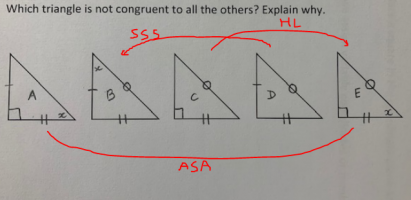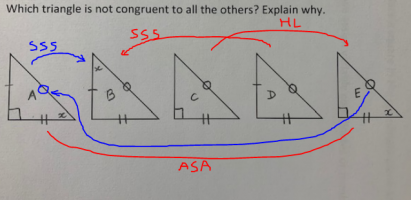Congruent triangles

Subhotosh Khan

Super Moderator
Staff member
Have you learned the theorems related to triangle congruence?

What are those?

Dr.Peterson

Elite Member
Please explain the notation. In particular, what does a circle on an edge mean? This is one of several things we would know if you had followed instructions and told us your thoughts about the problem:

Also, as drawn, all five triangles are congruent; presumably the question means to ask which one is not necessarily congruent to the others, based only on the markings. I'm not very happy with the statement of the problem!

But it's interesting that B and E are, respectively, D and C with an extra marking. This should be a useful clue (if it can be solved at all).

pearl12970

New member
Please explain the notation. In particular, what does a circle on an edge mean? This is one of several things we would know if you had followed instructions and told us your thoughts about the problem:

Also, as drawn, all five triangles are congruent; presumably the question means to ask which one is not necessarily congruent to the others, based only on the markings. I'm not very happy with the statement of the problem!

But it's interesting that B and E are, respectively, D and C with an extra marking. This should be a useful clue (if it can be solved at all).
Dr. Peterson, thank you so much for answering my question. My apologies for the confusion. My textbook uses the circle as one the ways to congruent sides.
I was able to see A is congruent to E by ASA, B congruent to D by SSS, C congruent to E by HL. But I am not able to figure out how C and D are congruent. Would really appreciate your help.

Thank you again!

pearl12970

New member
Have you learned the theorems related to triangle congruence?

What are those?
I have learned the theorems. SSS, SAS, ASA, AAS, HL.

pearl12970

New member
Please explain the notation. In particular, what does a circle on an edge mean? This is one of several things we would know if you had followed instructions and told us your thoughts about the problem:

Also, as drawn, all five triangles are congruent; presumably the question means to ask which one is not necessarily congruent to the others, based only on the markings. I'm not very happy with the statement of the problem!

But it's interesting that B and E are, respectively, D and C with an extra marking. This should be a useful clue (if it can be solved at all).
Sometimes, our teacher asks us questions where the answer is "no solution" so this is probably one of them, since all are congruent. Would really appreciate if you could please give me a hint for how C is congruent to D. I am not able to see which theorem applies to prove they are congruent.

pka

Elite Member
Please explain the notation. In particular, what does a circle on an edge mean? This is one of several things we would know if you had followed instructions and told us your thoughts about the problem:
I too found this to be an extremely irritating question. About the [imath]\circ[/imath], some fifty years while visiting my wife on one of her art exchanges I saw that used to indicate a third side of two triangle being congruent(in Africa or India)
So in this case[imath]\Delta(B)\cong \Delta(D)\text{ by }SSS[/imath]. Likewise [imath]\Delta(A)\cong \Delta(E)\text{ by }ASA[/imath]
I truly hope that peral12970 will answer with come clarification.

HallsofIvy

Elite Member
As long as sides of two triangles are marked the same way, where it is "|" or "||" or "o" I would assume they are congruent.

•pearl12970

pearl12970

New member
As long as sides of two triangles are marked the same way, where it is "|" or "||" or "o" I would assume they are congruent.
That’s correct. The circles indicate congruent sides. This question is straight out of a math textbook.

pearl12970

New member
I too found this to be an extremely irritating question. About the [imath]\circ[/imath], some fifty years while visiting my wife on one of her art exchanges I saw that used to indicate a third side of two triangle being congruent(in Africa or India)
So in this case[imath]\Delta(B)\cong \Delta(D)\text{ by }SSS[/imath]. Likewise [imath]\Delta(A)\cong \Delta(E)\text{ by }ASA[/imath]
I truly hope that peral12970 will answer with come clarification.
I am sorry you found this irritating. This is from my math textbook. Now imagine how we students might feel!

Dr.Peterson

Elite Member
Sometimes, our teacher asks us questions where the answer is "no solution" so this is probably one of them, since all are congruent. Would really appreciate if you could please give me a hint for how C is congruent to D. I am not able to see which theorem applies to prove they are congruent.
The first thing we can say is that if any four are congruent, then all five are, because they form two groups of congruent triangles, A=C=E and B = D:If the two groups are not congruent, then no triangle is different from all the others.

So the answer has to be "no solution", one way or the other.

But we can in fact go further, by considering all the triangles together, and realizing that the three markings represent the same values everywhere. From E, we can label the hypotenuse in A as O, so that A is congruent to B and D by SSS. Now we know that all five are congruent. You don't need to be able to directly relate every pair (though perhaps we could).Incidentally, we now know what x is as well.

It took me a while to figure this out, and I could still be wrong somewhere.

•pearl12970